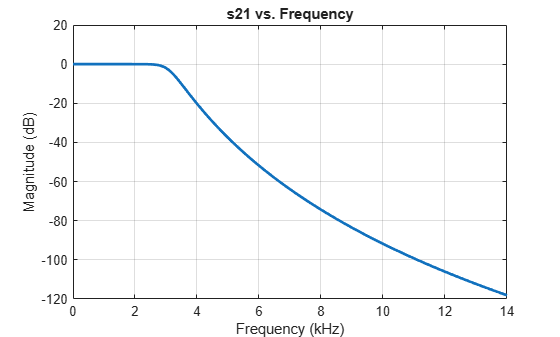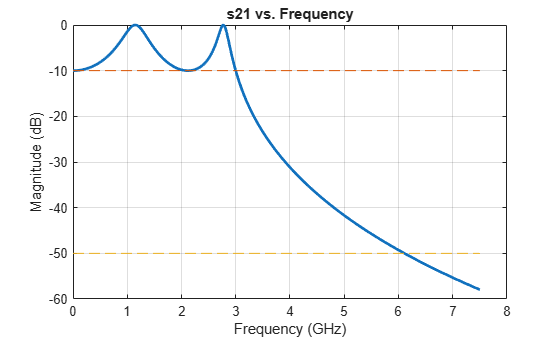# rffilter

Create Butterworth, Chebyshev, and inverse Chebyshev RF filter

## Description

Use the `rffilter` object to create a Butterworth, Chebyshev or an Inverse Chebyshev RF filter. The RF filter is a two-port circuit object, and you can include this object as an element of a circuit. For more design information see, Parameters to Define Filter and Design Tips.

You can also convert the `rffilter` object to a LC ladder filter by using the `lcladder` object. For example, `LCLad = lcladdder(rffiltobj)` where `rffiltobj` is an `rffilter` object.

## Creation

### Syntax

``rffiltobj = rffilter``
``rffiltobj = rffilter(Name=Value)``

### Description

example

````rffiltobj = rffilter` creates a two-port filter with default properties.```

example

````rffiltobj = rffilter(Name=Value)` sets properties using one or more name-value pairs. For example, ```rffiltobj = rffilter(FilterType='Chebyshev')``` creates a two-port Chebyshev RF filter. ```

## Properties

expand all

Filter type, specified as `'Butterworth'`, `'Chebyshev'`, or `'InverseChebyshev'`.

Example: `'FilterType','Chebyshev'`

Example: `rfobj.FilterType = 'Chebyshev'`

Data Types: `char` | `string`

Filter response type, specified as `'Lowpass'`, `'Highpass'`, `'Bandpass'`, or `'Bandstop'`. For more information, see Frequency Responses.

Example: `'ResponseType','Highpass'`

Example: `rfobj.ResponseType = 'Highpass'`

Data Types: `char` | `string`

Filter implementation, specified as `'LC Tee'`, `'LC Pi'`, or ```'Transfer function'```.

Example: ```'Implementation','Transfer function'```

Example: `rfobj.Implementation = 'Transfer function'`

#### Dependencies

For `'Inverse Chebyshev'` type filter, you can only use `'Transfer function'` implementation.

Data Types: `char` | `string`

Filter order, specified as a real finite non-negative integer. In a lowpass or highpass filter, the order specifies the number of lumped storage elements. In a bandpass or bandstop filter, the number of lumped storage elements is twice the value of the order.

Note

`FilterOrder` has the highest precedence among all the name-value pairs in the filter design. Using this property sets the `UseFilterOrder` read-only property to true.

Example: `'FilterOrder',4`

Example: `rfobj.FilterOrder = 4`

Data Types: `double`

Passband frequency, specified as:

• A scalar in hertz for lowpass and highpass filters.

• A two-element vector in hertz for bandpass or bandstop filters.

By default, the values are `1e9` for lowpass filter, `2e9` for highpass filter, and ```[2e9 3e9]``` for bandpass and `[[1e9 4e9]` for bandstop filters.

Example: `'PassbandFrequency',[3e6 5e6]`

Example: ```rfobj.PassbandFrequency = [3e6 5e6]```

Data Types: `double`

Stopband frequency, specified as:

• A scalar in hertz for lowpass and highpass filters.

• A two-element vector in hertz for bandpass or bandstop filters.

By default, the values are `2e9` for lowpass filter, `1e9` for highpass filter, ```[1.5e9 3.5e9]``` for bandpass filters, and ```[2.1e9 2.9e9]``` bandstop filters.

Example: ```rffilter('ResponseType','lowpass','StopbandFrequency',[3e6 5e6])```

Example: ```rfobj.StopbandFrequency = [3e6 5e6]```

Data Types: `double`

Passband attenuation, specified as a scalar in dB. For bandpass filters, this value is applied equally to both edges of the passband.

Example: `'PassbandAttenuation',5`

Example: `rfobj.PassbandAttenuation = 5`

Data Types: `double`

Stopband attenuation, specified as a scalar in dB. For bandstop filters, this value is applied equally to both edges of the stopband.

Example: `'StopbandAttenuation',30`

Example: `rfobj.StopbandAttenuation = 30`

Data Types: `double`

Source impedance, specified as a positive real part finite scalar in ohms.

Example: `'Zin',70`

Example: `rfobj.Zin = 70`

Data Types: `double`

Load impedance, specified as a positive real part finite scalar in ohms.

Example: `'Zout',70`

Example: `rfobj.Zout = 70`

Data Types: `double`

Name of RF filter object, specified as a character vector. Two elements in the same circuit cannot have the same name. All names must be valid MATLAB® variable names.

Example: `'Name','filter1'`

Example: `rfobj.Name = 'filter1'`

Data Types: `char` | `string`

Number of ports, specified as a `2`. This property is read-only.

Data Types: `double`

Names of the terminals, specified as a {'p1+','p2+','p1-','p2-'}. This property is read-only.

Data Types: `char`

Filter design data, specified as a structure. This property is read-only. For more information, see Design Data for LC Tee and LC Pi Topologies and Design Data for Transfer Function Implementation.

Data Types: `struct`

Use of filter order for filter design, specified as a `true` or `false`. This property is a read-only.

Data Types: `logical`

## Object Functions

 `groupdelay` Group delay of S-parameter object or RF filter object or RF Toolbox circuit object `sparameters` Calculate S-parameters for RF data, network, circuit, and matching network objects `set` Set `rffilter` object property values `zpk` Converts rffilter to zero-pole-gain representation `tf` Converts rffilter to transfer function `lcladder` Create LC ladder network `rfplot` Plot input reflection coefficient and transducer gain of matching network `clone` Create copy of existing circuit element or circuit object `circuit` Circuit object

## Examples

collapse all

Create and view the properties of a default RF filter object.

`rfobj = rffilter`
```rfobj = rffilter: Filter element FilterType: 'Butterworth' ResponseType: 'Lowpass' Implementation: 'LC Tee' FilterOrder: 3 PassbandFrequency: 1.0000e+09 PassbandAttenuation: 3.0103 Zin: 50 Zout: 50 DesignData: [1x1 struct] UseFilterOrder: 1 Name: 'Filter' NumPorts: 2 Terminals: {'p1+' 'p2+' 'p1-' 'p2-'} ```
`rfobj.DesignData`
```ans = struct with fields: FilterOrder: 3 Inductors: [7.9577e-09 7.9577e-09] Capacitors: 6.3662e-12 Topology: 'lclowpasstee' PassbandFrequency: 1.0000e+09 PassbandAttenuation: 3.0103 ```

Create a Butterworth passband filter object named `BFCG_162W` with passband frequencies between 950 and 2200 MHz, stopband frequencies between 770 and 3000 MHz, passband attenuation of 3.0 dB, and stopband attenuation of 40 dB using 'LC Tee' implementation type. Calculate the S-parameters of the filter at 2.1 GHz.

```robj = rffilter('ResponseType','Bandpass','Implementation','LC Tee','PassbandFrequency',[950e6 2200e6], ... 'StopbandFrequency',[770e6 3000e6],'PassbandAttenuation',3,'StopbandAttenuation',40); robj.Name = 'BFCG_162W'```
```robj = rffilter: Filter element FilterType: 'Butterworth' ResponseType: 'Bandpass' Implementation: 'LC Tee' PassbandFrequency: [950000000 2.2000e+09] PassbandAttenuation: 3 StopbandFrequency: [770000000 3.0000e+09] StopbandAttenuation: 40 Zin: 50 Zout: 50 DesignData: [1x1 struct] UseFilterOrder: 0 Name: 'BFCG_162W' NumPorts: 2 Terminals: {'p1+' 'p2+' 'p1-' 'p2-'} ```

Calculate the S-parameters at 2.1 GHz.

`s = sparameters(robj,2.1e9)`
```s = sparameters: S-parameters object NumPorts: 2 Frequencies: 2.1000e+09 Parameters: [2x2 double] Impedance: 50 rfparam(obj,i,j) returns S-parameter Sij ```

Build a `lcladder` object from the `rffilter` object. This lcladder object can be used in a circuit directly and could also be used for parametric analysis across inductor and capacitance values.

`l = lcladder(robj)`
```l = lcladder: LC Ladder element Topology: 'bandpasstee' Inductances: [1.8116e-09 5.7297e-09 8.3361e-09 2.8294e-09 1.2214e-08 2.3802e-09 1.2214e-08 2.8294e-09 8.3361e-09 5.7297e-09 1.8116e-09] Capacitances: [6.6900e-12 2.1152e-12 1.4539e-12 4.2835e-12 9.9228e-13 5.0919e-12 9.9228e-13 4.2835e-12 1.4539e-12 2.1152e-12 6.6900e-12] Name: 'lcfilt' NumPorts: 2 Terminals: {'p1+' 'p2+' 'p1-' 'p2-'} ```

Alternatively, to access the inductors and capacitors directly from the filter object use:

```L = robj.DesignData.Inductors; C = robj.DesignData.Capacitors;```

Create a Chebyshev lowpass filter with a passband frequency of 2 GHz.

`robj = rffilter('FilterType','Chebyshev','PassbandFrequency',2e9);`

Set the filter order to `5` and the implementation to `LC Pi`.

`set(robj,'FilterOrder',5,'Implementation','LC Pi');`

Calculate the group delay of the filter at 1.9 GHz.

`groupdelay(robj,1.9e9)`
```ans = 1.4403e-09 ```

This example shows how to design a low-pass Butterworth filter with passband frequency of 3 kHz, stopband frequency 7 kHz, passband attenuation of 2 dB, and stopband attenuation 60 dB. Display the filter order of such a designed filter and determine the passband frequency at 3.0103 dB. See  in `rffilter` object page.

Filter Parameters

```Fp = 3e3; % Passband frequency, Hz Ap = 2; % Passband attenuation, dB Fs = 7e3; % Stopband frequency, Hz As = 60; % Stopband attenuation, dB```

Design Filter

```r = rffilter("FilterType","Butterworth","ResponseType","Lowpass","Implementation","Transfer function","PassbandFrequency",Fp, ... "PassbandAttenuation",Ap,"StopbandFrequency",Fs,"StopbandAttenuation",As);```

Filter Order of Designed Filter

```N = r.DesignData.FilterOrder; sprintf('Calculated filter order is %d',N)```
```ans = 'Calculated filter order is 9' ```

Frequency at 3.0103 dB

```F_3dB = r.DesignData.PassbandFrequency/1e3; sprintf('Frequency at 3.0103 dB is %d kHz',F_3dB)```
```ans = 'Frequency at 3.0103 dB is 3.090733e+00 kHz' ```

Visualize Magnitude Response

```frequencies = linspace(0,2*Fs,1001); rfplot(r, frequencies)```Note: To use `rfplot` and `plot` on the same figure use `setrfplot`. Type `'help setrfplot`' in command window for information.

Reference

1. Larry D. Paarmann, Design and Analysis of Analog Filters: A Signal Processing Perspective, Kluwer Academic Publishers

Design a low-pass Chebyshev filter with 0.1 dB bandpass ripple, cut-off frequency of 1 rad/sec, and 50 dB attenuation at 1.1 rad/sec. Display the filter order of this designed filter .

Define Parameters

```Fp = 1/(2*pi); % Passband frequency, Hz Rp = 0.1; % Ripple in Passband, dB Fs = 1.1/(2*pi); % Stopband frequency, Hz As = 50; % Stopband attenuation, dB```

Design Filter

```r = rffilter("FilterType","Chebyshev","ResponseType","Lowpass","Implementation","Transfer function","PassbandFrequency",Fp, ... "PassbandAttenuation",Rp,"StopbandFrequency",Fs,"StopbandAttenuation",As)```
```r = rffilter: Filter element FilterType: 'Chebyshev' ResponseType: 'Lowpass' Implementation: 'Transfer function' PassbandFrequency: 0.1592 PassbandAttenuation: 0.1000 StopbandFrequency: 0.1751 StopbandAttenuation: 50 Zin: 50 Zout: 50 DesignData: [1x1 struct] UseFilterOrder: 0 Name: 'Filter' NumPorts: 2 Terminals: {'p1+' 'p2+' 'p1-' 'p2-'} ```

Filter Order of Designed Filter

```N = r.DesignData.FilterOrder; sprintf('Calculated filter order is %d',N)```
```ans = 'Calculated filter order is 19' ```

Reference

1. G.Ellis, Michael,Sr.Electronic Filter Analysis and Synthesis,Artech House, 1994

Design even order Chebyshev filter and plot the filter's frequency response.

Frequency Response of Even Order Chebyshev Filter using Transfer Function Implementation

Design even order Chebyshev filter with specified parameters.

```Fp = 3e9; % Passband frequency, GHz Rp = 10; % Passband attenuation, dB Fs = 7e9; % Stopband frequency, GHz As = 50; % Stopband attenuation, dB rffiltobj = rffilter("FilterType","Chebyshev","ResponseType","Lowpass","Implementation","Transfer function","PassbandFrequency",Fp,... "PassbandAttenuation",Rp,"StopbandAttenuation",As, "StopbandFrequency",Fs);```

Plot the frequency response of even order Chebyshev filter using `rfplot` function.

`rfplot(rffiltobj,linspace(0,7.5e9,1001))`

Visualize the stopband and passband attenuation using `plot` function.

```hold on; plot([0 7.5], repmat([-rffiltobj.PassbandAttenuation -rffiltobj.StopbandAttenuation],2,1),'--')```Use `DesignData` parameter to verify the order of your filter.

```N = rffiltobj.DesignData.FilterOrder; sprintf('Calculated filter order is %d',N)```
```ans = 'Calculated filter order is 4' ```